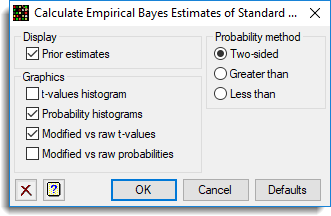1. Home
2. Empirical Bayes Estimates Options

# Empirical Bayes Estimates Options

Use this to to specify options for an empirical Bayes error estimation analysis.## Display prior estimates

Display the estimates of the prior parameters, d0 and s0, in the Output window.

## Probability method

This lets you choose the type of t-test to use for the probabilities:

 Two-sided The probability is calculated as P(-|T| <= t <= |T|) Greater than The probability is calculated as P(t >= T) Less than The probability is calculated as P(t <= T)

## Graphics

Controls which graphics are to be displayed with the analysis:

 t-values histogram Two histograms showing the modified and raw t-values plotted on the same scale. Probability histogram Two histograms showing the modified and raw probabilities plotted on the same scale. Modified vs raw t-values A scatter plot of modified t-values versus raw t-values. Modified vs raw probabilities A scatter plot of modified probabilities versus raw probabilities.

## Action buttons

 OK Save the options settings and close the dialog. Cancel Close the dialog without making any changes. Defaults Reset options to their default settings.

## Action IconsClear Clear all fields and list boxes.Help Open the Help topic for this dialog.